Function Repository Resource:

# CrossNodeGridGraph

Contributed by: Utkarsh Patel and Simon Fischer
 ResourceFunction["CrossNodeGridGraph"][r,d] creates a d-dimensional grid graph with r cross-linked vertices in all dimensions. ResourceFunction["CrossNodeGridGraph"][{n1,n2,…,nd}] creates a d-dimensional grid graph with ni cross-linked vertices in each dimension.

## Details and Options

ResourceFunction["CrossNodeGridGraph"] can be used to create fractional dimension hypergraphs.
ResourceFunction["CrossNodeGridGraph"] has the same options as Graph, with the following additions and changes:
 "CoordinateLabeled" True whether to change vertex labels to a Cartesian coordinate system with the central vertex as origin VertexLabels Automatic labels and placements for vertices VertexSize Tiny size of vertices
With the setting , the vertices are laid out in an orthogonal grid for dimensions 2 and 3.

## Examples

### Basic Examples (2)

 In:=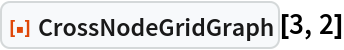Out=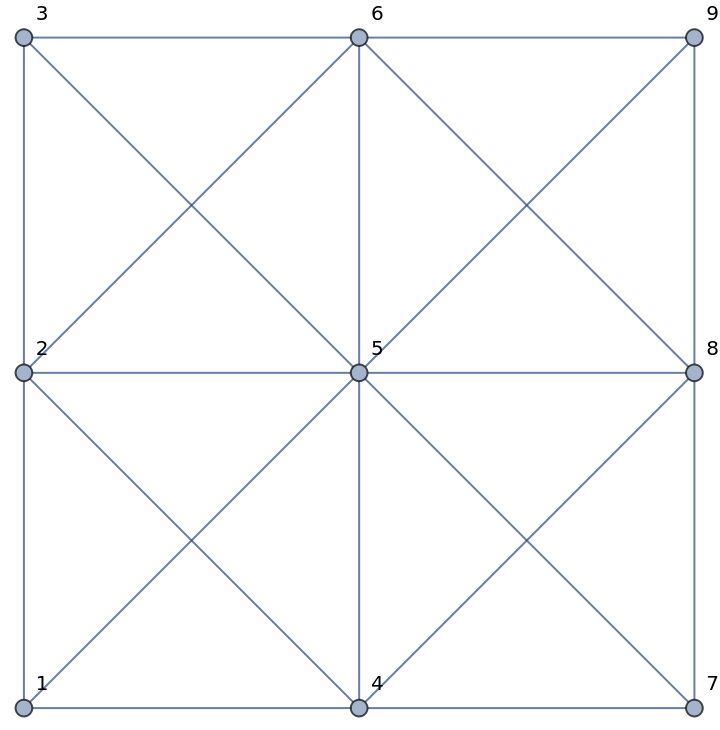In:=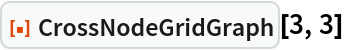Out=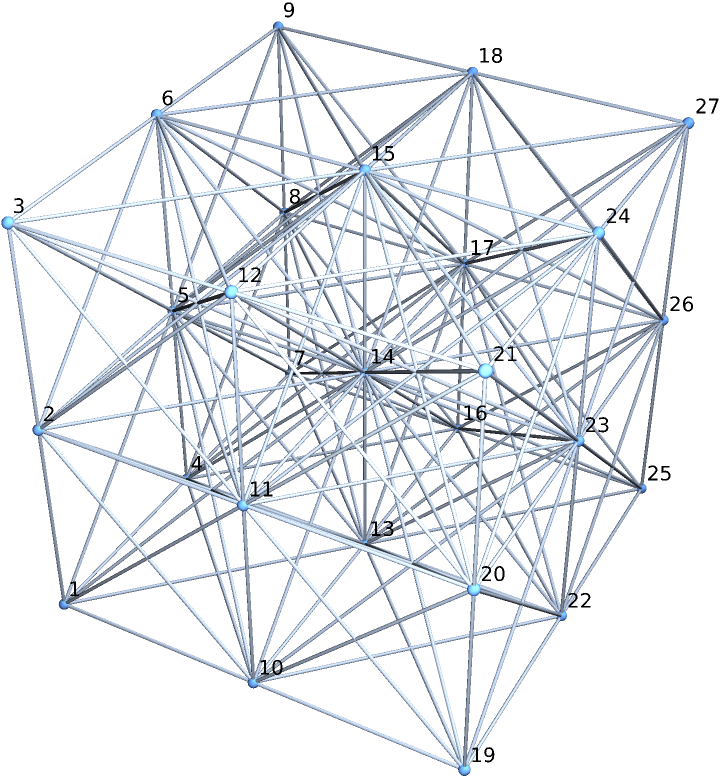### Scope (2)

A 5-dimensional cross-linked grid graph, embedded in 3D:

 In:=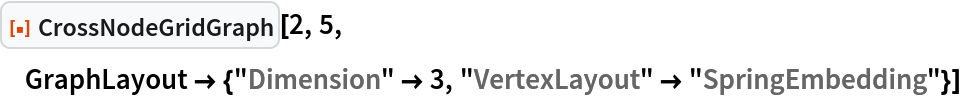Out=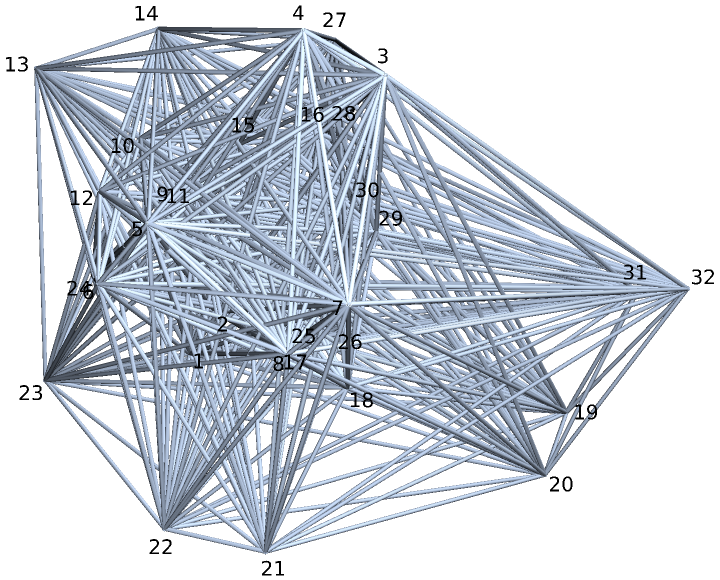A 2-dimensional rectangular cross-linked grid graph:

 In:=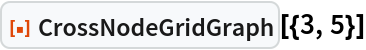Out=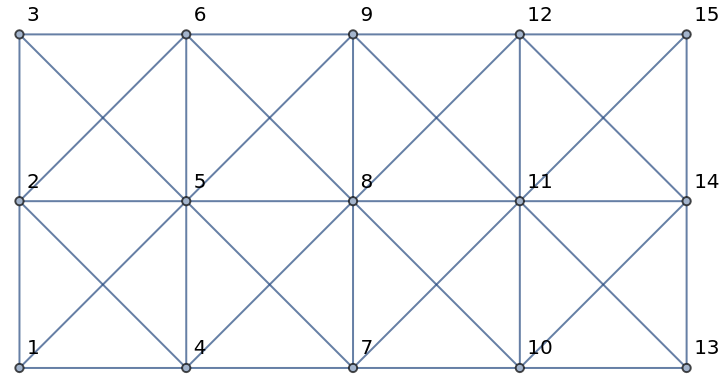### Options (2)

#### CoordinateLabeled (2)

With "CoordinateLabeled"->False, the vertex labels are set as their indices:

 In:=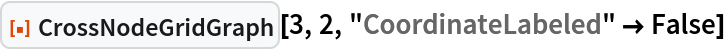Out=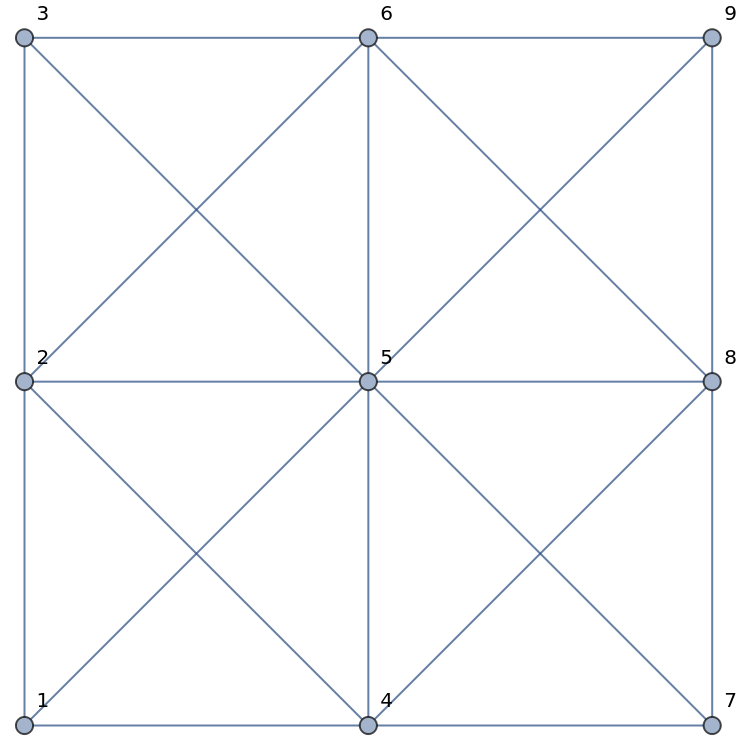With "CoordinateLabeled"->True, the vertex labels are set as their Cartesian coordinates:

 In:=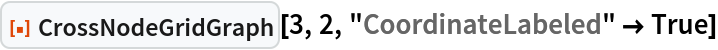Out=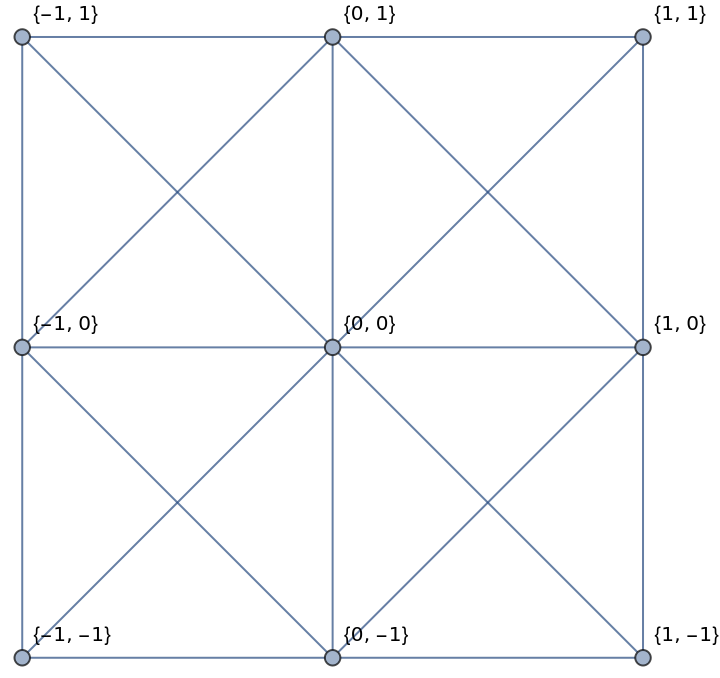A 3-dimensional cross-linked grid graph with vertices labeled as their Cartesian coordinates:

 In:=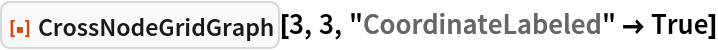Out=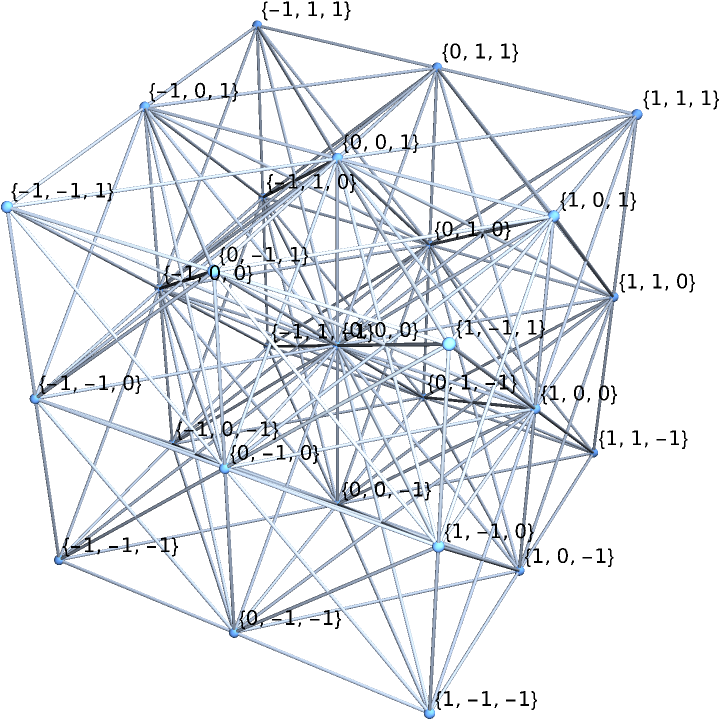### Properties and Relations (3)

CrossNodeGridGraph[r,d] is equivalent to CrossNodeGridGraph[ConstantArray[r,d]]:

 In:=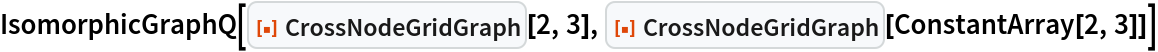Out=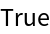The tetrahedral graph is a special case of CrossNodeGridGraph:

 In:=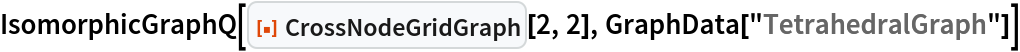Out=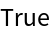CompleteGraph is a special case of CrossNodeGridGraph:

 In:=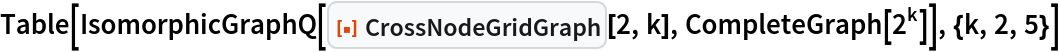Out=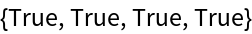### Neat Examples (2)

Visualize a perspective projection of a 4D cross-linked grid graph:

 In:=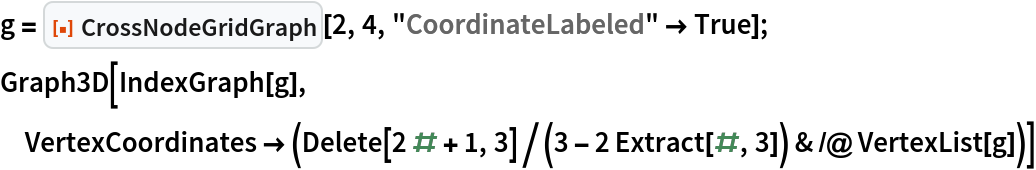Out=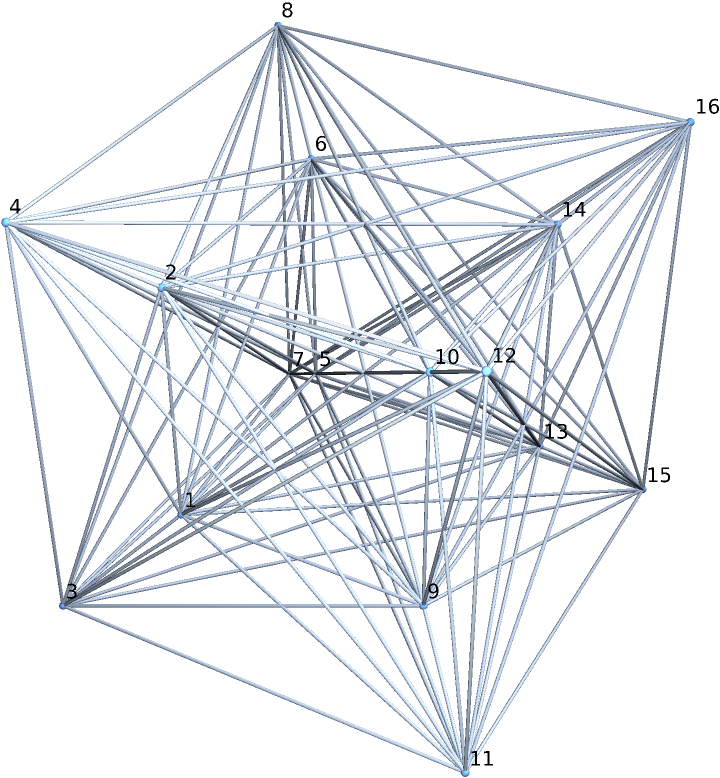Create a 2D cross-linked grid graph:

 In:=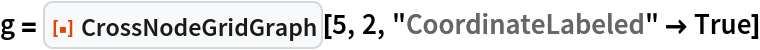Out=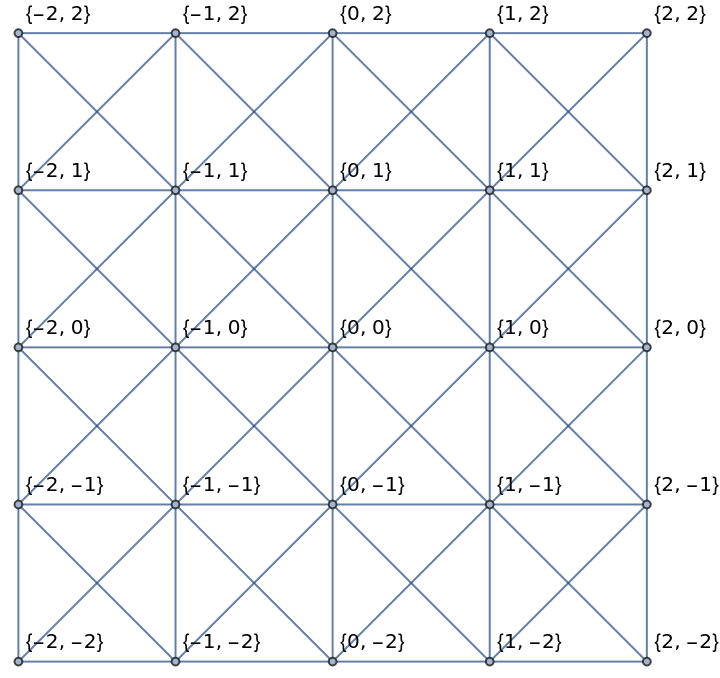The resource function WolframHausdorffDimension can be used to verify that the dimensionality of the graph's vertices are of fractional order:

 In:=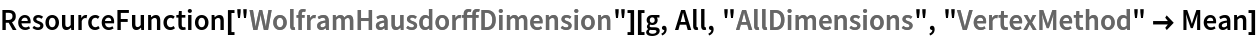Out=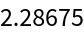In:=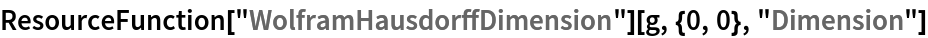Out=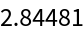Utkarsh Patel

## Version History

• 1.0.0 – 25 July 2022